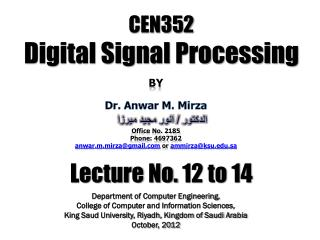Download PresentationCEN352 Digital Signal Processing

# CEN352 Digital Signal Processing

Download Presentation## CEN352 Digital Signal Processing

- - - - - - - - - - - - - - - - - - - - - - - - - - - E N D - - - - - - - - - - - - - - - - - - - - - - - - - - -
##### Presentation Transcript

1. By Dr. Anwar M. Mirza Office No. 2185 Phone: 4697362 anwar.m.mirza@gmail.com or ammirza@ksu.edu.sa CEN352Digital Signal Processing الدكتور / انور مجيد ميرزا Lecture No. 12 to 14 Department of Computer Engineering, College of Computer and Information Sciences, King Saud University, Riyadh, Kingdom of Saudi Arabia October, 2012

2. It is a very efficient algorithm for computing the DFT coefficients. Fast Fourier Transform

3. z=x+y x x z=wx z=x - y x y y w -1 Definitions of the graphical operations Method of Decimation-in-Frequency a(0) x(0) X(0) (N/2)-point DFT a(1) X(2) x(1) a(2) X(4) x(2) a3) X(6) x(3) WN0 b(0) -1 x(4) X(1) (N/2)-point DFT WN1 b(1) -1 x(5) X(3) WN2 -1 b(2) X(5) x(6) WN3 -1 b(3) x(7) X(7) The first iteration of the 8-point FFT

4. Method of Decimation-in-Frequency (N/4)-point DFT (N/4)-point DFT (N/4)-point DFT X(0) x(0) X(2) x(1) (N/4)-point DFT X(4) x(2) WN0 x(3) X(6) WN0 -1 -1 WN2 X(1) x(4) WN1 -1 -1 WN0 x(5) X(3) The second iteration of the 8-point FFT WN2 -1 -1 WN2 x(6) X(5) WN3 -1 -1 x(7) X(7)

5. Method of Decimation-in-Frequency WN0 WN0 X(0) x(0) -1 -1 WN0 x(1) X(2) X(4) x(2) -1 -1 WN0 x(3) X(6) WN0 -1 -1 x(4) X(1) WN1 -1 -1 WN0 x(5) X(3) Block diagram for the 8-point FFT (total twelve multiplications) WN2 -1 -1 WN2 x(6) X(5) WN3 -1 -1 x(7) X(7)

6. Example 4.12 x(0)=1 Solution x(1)=2 Bit Reversal Bit Index x(2)=3 10 00 00 = X(0) First Iteration Second Iteration x(3)=4 01 10 -2 = X(2) 4 10 -2+2j 01 10 = X(1) 6 -2 11 -2-2j 11 = X(3) W40 = 1 -2+2j -2 -2 W40 = 1 -2-2j -2 2j W41 = -j W40 = 1

7. Example 4.13 X(0)=10 Solution X(1)=-2+j2 Bit Reversal Bit Index X(2)=-2 4 00 00 = x(0) First Iteration Second Iteration X(3)=-2-j2 12 10 01 = x(2) 8 10 8 10 01 = x(1) -4 -2 11 11 16 = x(3) -2+2j 12 12 -2-2j j4 -4

8. Method of Decimation-in-Time The first iteration of the 8-point FFT using Decimation-in-Time

9. Method of Decimation-in-Time The second iteration of the 8-point FFT using Decimation-in-Time

10. Method of Decimation-in-Time The 8-point FFT using Decimation-in-Time (12 complex multiplications)

11. Method of Decimation-in-Time The 8-point IFFT using Decimation-in-Time

12. The 4-point FFT using Decimation-in-Time

13. The 4-point FFT using Decimation-in-Time

14. That’s it for today !!

15. Example 4.12 x(0)=6 Solution x(1)=4 Bit Reversal Bit Index x(2)=2 12 00 00 = X(0) First Iteration Second Iteration x(3)=0 01 10 4 = X(2) 8 12 4-4j 01 10 = X(1) 4 4 11 4+4j 11 = X(3) W40 = 1 4-4j 4 4 W40 = 1 4+4j 4 -4j W41 = -j W40 = 1

16. Example 4.12 x(0)=1 Solution x(1)=2 Bit Reversal Bit Index x(2)=3 10 00 00 = X(0) First Iteration Second Iteration x(3)=4 01 10 -2 = X(2) 4 10 -2+2j 01 10 = X(1) 6 -2 11 -2-2j 11 = X(3) W40 = 1 -2+2j -2 -2 W40 = 1 -2-2j -2 2j W41 = -j W40 = 1